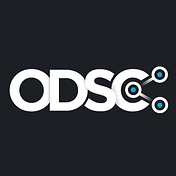# What you’ll get out of this post and what you’ll need to run the code

## Step 1: Load data

`import sklearn.datasetsimport sklearn.model_selection# We fetch the data using openml.orgX, y = sklearn.datasets.fetch_openml(data_id=31, return_X_y=True, as_frame=True)# Split the data into train and testX_train, X_test, y_train, y_test = sklearn.model_selection.train_test_split(   X, y, test_size=0.4, random_state=42)X_train.info()`

## Step 2: Manually build a pipeline

`from sklearn.compose import ColumnTransformerfrom sklearn.metrics import accuracy_scorefrom sklearn.pipeline import Pipelinefrom sklearn.preprocessing import OneHotEncoderfrom sklearn.preprocessing import StandardScalerfrom sklearn.svm import SVC# Create the estimator using the default parameters from the libraryestimator_svc = SVC(C=1.0, kernel='rbf', gamma='scale', shrinking=True, tol=1e-3,   cache_size=200, verbose=False, max_iter=-1, random_state=42)# build and fit the pipelinecategorical_columns = [col for col in X_train.columns                      if X[col].dtype.name == 'category']encoder = ColumnTransformer(transformers = [ ('cat', OneHotEncoder(handle_unknown='ignore'), categorical_columns)], remainder='passthrough')pipeline_svc = Pipeline([ ('encoder', encoder), ('scaler', StandardScaler()), ('svc', estimator_svc),])pipeline_svc.fit(X_train, y_train)`
`# Score the modelprediction = pipeline_svc.predict(X_test)performance_svc = accuracy_score(y_test, prediction)print(f"SVC performance is {performance_svc}")`

## Step 3: Use Auto-sklearn as a drop-in-replacement

`import autosklearn.classification# Create and train the estimatorestimator_askl = autosklearn.classification.AutoSklearnClassifier(   time_left_for_this_task=300,   seed=42,   resampling_strategy='cv',   n_jobs=1,)estimator_askl.fit(X_train, y_train)# Score the modelprediction = estimator_askl.predict(X_test)performance_askl = accuracy_score(y_test, prediction)print(f"Auto-Sklearn Classifier performance is {performance_askl}")`

# Wrapping up on Auto-sklearn

--

--## ODSC - Open Data Science

Our passion is bringing thousands of the best and brightest data scientists together under one roof for an incredible learning and networking experience.